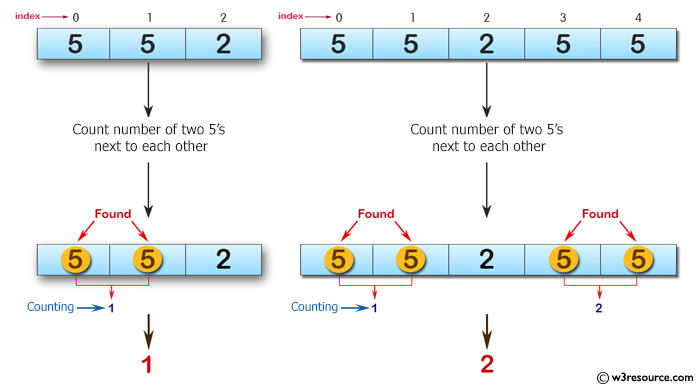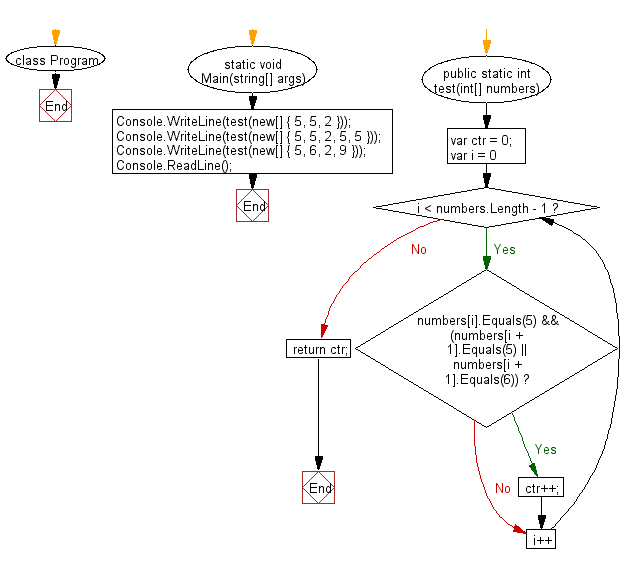﻿ C# - Find the number of 5s next to each other in an array# C# Sharp Basic Algorithm Exercises: Count the number of two 5's are next to each other in an array of integers

## C# Sharp Basic Algorithm: Exercise-38 with Solution

Write a C# Sharp program to count the number of two 5's are next to each other in an array of integers. Also count the situation where the second 5 is actually a 6.

Pictorial Presentation:Sample Solution:-

C# Sharp Code:

``````using System;
using System.Linq;
namespace exercises
{
class Program
{
static void Main(string[] args)
{
Console.WriteLine(test(new[] { 5, 5, 2 }));
Console.WriteLine(test(new[] { 5, 5, 2, 5, 5 }));
Console.WriteLine(test(new[] { 5, 6, 2, 9 }));
}
public static int test(int[] numbers)
{
var ctr = 0;
for (var i = 0; i < numbers.Length - 1; i++)
{
if (numbers[i].Equals(5) && (numbers[i + 1].Equals(5) || numbers[i + 1].Equals(6))) ctr++;
}
return ctr;
}
}
}
```
```

Sample Output:

```1
2
1```

Flowchart:C# Sharp Code Editor:

Improve this sample solution and post your code through Disqus

What is the difficulty level of this exercise?

Test your Programming skills with w3resource's quiz.

﻿